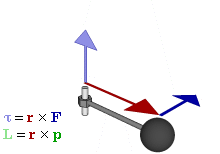#Difference Between Similar Terms and Objects

# Difference Between Torque and MomentDemonstrating relationship between force (F), torque (τ), linear momentum (p), angular momentum (L), and position (r) of rotating particle.

Torque vs Moment

Motion is defined as a change in the position of an object. It can be described in terms of velocity, speed, acceleration, direction, displacement, shape, and time. Force is necessary to change the position of an object as it is the influence that causes an object to undergo changes.

The force can either be a push or a pull which causes an object to change its form and speed. Many forces can be applied to objects, two of which are torque and moment. In physics, they may refer to the same thing, but in mechanical engineering they are different.

Moment is a concept of physics and engineering that refers to the tendency of a force to move an object. It is a measure of the tendency of the force to rotate an object at an axis through a point. It is the perpendicular distance from the point of rotation to the force’s line of action.

It can be calculated at any point, and its value is the result of the force and the moment arm. Its symbol is the letter “M,” and the Newton meter (Nm) is its International System of Units (SI) unit. It is represented as “NM.”
Torque, on the other hand, can be synonymous with moment. It refers to the tendency of a force to cause the rotation of an object in an axis or a pivot. It is the measure of the turning force of an object, and it is used to measure coupling.
When two equal and opposite forces act together and cause an object to rotate or turn, there is coupling. An example is the force applied by the hand on a screwdriver. This moment of a coupling is called a torque. Its symbol is the Greek letter “tau,” and its International System of Units (SI) unit is also the Newton meter, the same as moment. It is presented as “Nm/revolution” and is an application of moment.
While moment is a static force, is produced by any lateral force, and is used in non-rotational circumstances, torque is a movement force and is used when there is a pivot.

Summary:

1.“Moment” is a concept of engineering and physics that refers to the tendency of a force to move an object while torque is the tendency of a force to rotate an object in a pivot.
2.Moment is the perpendicular distance between the point of rotation and the force’s line of action while torque is a measure of the turning force of an object.
3.Torque is used to measure coupling while moment is not.
4.Both torque and moment have the Newton meter as the SI unit; torque is presented as Nm/revolution while moment is presented as Nm.
5.Moment is a static force while torque is a movement force.
6.Moment is used when there is no rotation while torque is used when there is rotation and a pivot.
7.Moment has the symbol “M” while torque has the Greek letter “tau” as its symbol.

### Search DifferenceBetween.net :

Custom Search

Help us improve. Rate this post!(6 votes, average: 3.17 out of 5)Loading...Email This Post : If you like this article or our site. Please spread the word. Share it with your friends/family.

1. Torque is perpendicular on F & r both.

3. “Force is necessary to change the position of an object as it is the influence that causes an object to undergo changes.” Not true. If it is in motion it will remain in motion. Force is necessary to change the momentum of the object.

4. Nice explanation